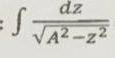# Kinematics: find the particle's position as a function of time

## Homework Statement

There is a particle that moves in 1-dimension. mass is m, force on the object is given as a function of position(x), Fx= -mω2x.(ω is constant) when t=0 particle has x0>0, and velocity is zero. find the particle's position as a function of time.

## Homework Equations

There is a given hint for the problem. Use z=Acosu conversion on this integral## The Attempt at a Solution

What i did so far: I used Newton's second law so that i can find accerelation. Then i integrate accerelation function and find velocity function as a function of position. Lastly i integrate velocity function and i find x= x02x3/6. From there i can't do anything. I couldn't use motion equations because accerelation is not constant. And i couldn't find how to use this integral.

#### Attachments

haruspex
Homework Helper
Gold Member
What i did so far:
Please post your working, or we have little idea where you are going wrong.
(It looks like you have the wrong idea about how to solve differential eauations. You cannot integrate ∫f(x).dt as though it were ∫f(x).dx.)

Please post your working, or we have little idea where you are going wrong.
(It looks like you have the wrong idea about how to solve differential eauations. You cannot integrate ∫f(x).dt as though it were ∫f(x).dx.)
Yes you are right. If i didn't make a mistake i find x= x02xt2/2

haruspex
Homework Helper
Gold Member
Yes you are right. If i didn't make a mistake i find x= x02xt2/2
No, you can't do that either. That is treating x as a constant.
I don't uderstand why the hint quotes that integral, but the substitution it recommends should help. Try x=A cos(u).

Orodruin
Staff Emeritus
•